# Overview

ggloop’s main function is ggloop(), and it allows the user to create one of two things:

1. Multiple ggplot2 plots.
2. A list of aesthetics, which can used to make ggplot2 plots using ggplot().

Instead of passing a single aesthetic (x, y, ...) to ggplot(), the user can pass multiple aesthetics by using a vector of aesthetics. “Remapping”" behaviors control how the x and y vectors are paired with one another, and they control how mutliple ... arguments are paired with one another.

The gg_obs argument controls the returned value: either ggplot2 plots or a list of aesthetics which can be used to make ggplot2 plots. gg_obs’s default value is TRUE, which instructs ggloop() to return plots. FALSE instructs ggloop() to return a list of aesthetics.

# Main Functions

## ggloop

Mimicry of ggplot()’s arguments (for the most part).

#### Args

• data = Same as data
• mappings = Same as mapping, except aes_loop() is used in place of aes(). There currently is no support for a “strings”-type function.
• remap_xy = Controls the “remapping” behavior of the x and y vectors. Default = TRUE; other options include FALSE and NA.
• TRUE = acts like expand.grid() except that exact duplicates (i.e. x <- mpg; y <- mpg) and unordered-pair duplicates (i.e. x <- mpg; y <- cyl and x <- cyl; y <- mpg) are removed.
• FALSE = acts like R’s internal recycling mechanism, which replicates the shorter of the two vectors however many times necessary to pair with the longer vector.
• NA = leaves an unpaired vector element unpaired (i.e. x = (mpg, wt, hp); y = (cyl, gear) produces x <- hp with no y mapping)
• remap_dots = Controls the “remapping” behavior of the dots arguments. Default = FALSE; other option is FALSE.
• TRUE = acts like R’s internal recycling mechanism, which replicates the shorter of the two vectors however many times necessary to pair with the longer vector.
• FALSE = leaves un unpaired vecotr element unpaired; similar to remap_xy = NA behavior.
• gg_obs = Controls the returned value. TRUE (default) returns ggplot2 plots; FALSE returns a list of aesthetics (see Value).
• ... = The same as ...; directly passed to ggplot().
• environment = The same as environment; directly passed into ggplot().

#### Value

There are two main types of returned values when gg_obs = TRUE:

1. A list of ggplot2 plots - when no ... are specified in aes_loop().
2. A nested list (a list of a list) of ggplot2 plots - when there are ... specified in aes_loop().

## aes_loop

Mimicry of ggplot()’s aes(), except:

1. You can use dplyr’s syntax to call variables (i.e. y = mpg:cyl, x = 1:5, etc). You can still use ggplot2’s syntax to call variables (i.e. x = disp, x = mpg/hp, color = factor(cyl), y = gear + cyl, etc). You can also use both within a vector, but do adhere to the rule below.
2. If you are calling more than one variable, then you need to wrap the variables in c() and not have any other c() within that wrapping (i.e. x = c(mpg:cyl, 5, 6:8, gear + cyl, mpg/hp), color = c(factor(cyl), factor(gear))).

#### Args

• x = Same as x
• y = Same as y
• ... = Same as ...

## %L+%

Mimicry of + with magrittr-like implementation. Can be used to add to three different types of objects:

1. A single ggplot2 plot.
2. A list of ggplot2 plots.
3. A nested list (a list of a list) of ggplot2 plots.

The user thus has the ability to add components to a subset of plots. Much like +, the results of %L+% will not be saved unless they are assigned.

# A Trivial Example

### Example 1

Example: Using the mtcars data set, create and plot all possible xy-pairs between mpg and hp.
Steps: Load the necessary packages, create plots with ggloop(), and add to plots using %L+%.

library(ggplot2)
library(ggloop)
g <- ggloop(mtcars, aes_loop(x = mpg:hp, y = mpg:hp))

View some of the plots.

g$x.mpg_y.hp %L+% geom_point()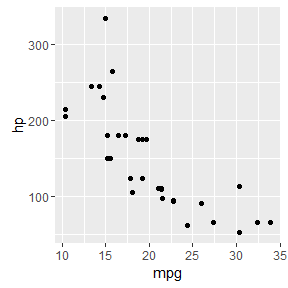g$x.cyl_y.disp %L+% geom_point()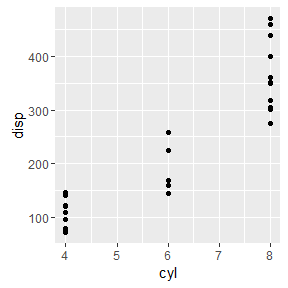g$x.disp_y.hp %L+% geom_point() %L+% geom_line()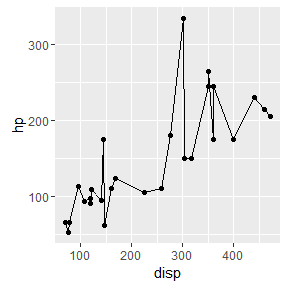Use %L+% to add to two plots, save the output, and then display the results. g[1:2] <- g[1:2] %L+% geom_point() g[1:2] ##$x.mpg_y.cyl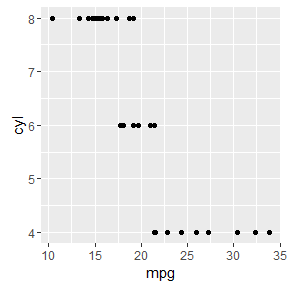##
## $x.mpg_y.disp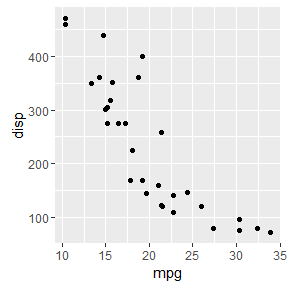Look at the mappings of each name in g. Note how the list is NOT nested (because no ... were called in aes_loop()). lapply(g, [[, "mapping") ##$x.mpg_y.cyl
## * x -> mpg
## * y -> cyl
##
## $x.mpg_y.disp ## * x -> mpg ## * y -> disp ## ##$x.mpg_y.hp
## * x -> mpg
## * y -> hp
##
## $x.cyl_y.disp ## * x -> cyl ## * y -> disp ## ##$x.cyl_y.hp
## * x -> cyl
## * y -> hp
##
## $x.disp_y.hp ## * x -> disp ## * y -> hp ### Example 2 Example: Plot c(mpg, disp, hp) against wt and facet the resulting plots. Steps: Create plots, add geoms, and add facet_grid(). g2 <- ggloop(mtcars, aes_loop(x = c(mpg, disp, hp), y = wt, color = factor(cyl))) %L+% geom_point() %L+% facet_grid(. ~ cyl) View some plots g2$color.factor(cyl)[1:3]
## $x.mpg_y.wt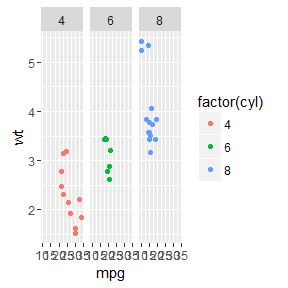## ##$x.disp_y.wt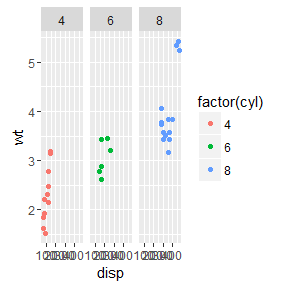##
## $x.hp_y.wt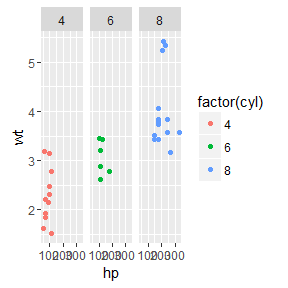Look at the mappings of each name in g2. Note how the list is nested (because a ... argument was called in aes_loop()). lapply(g2, function(x) lapply(x, [[, "mapping")) ##$color.factor(cyl)
## $color.factor(cyl)$x.mpg_y.wt
## * x      -> mpg
## * y      -> wt
## * colour -> factor(cyl)
##
## $color.factor(cyl)$x.disp_y.wt
## * x      -> disp
## * y      -> wt
## * colour -> factor(cyl)
##
## $color.factor(cyl)$x.hp_y.wt
## * x      -> hp
## * y      -> wt
## * colour -> factor(cyl)

### Example 3

Example: Return the list of aesthetics rather than the list of plots.
Steps: Call ggloop() with gg_obs = FALSE.

g3 <- ggloop(mtcars,
aes_loop(x = c(mpg, disp, hp), y = wt, color = c(factor(cyl), factor(gear))),
gg_obs = FALSE)
print(g3)
## []
## [][]
## * x      -> mpg
## * y      -> wt
## * colour -> factor(gear)
##
## [][]
## * x      -> disp
## * y      -> wt
## * colour -> factor(gear)
##
## [][]
## * x      -> hp
## * y      -> wt
## * colour -> factor(gear)
##
##
## []
## [][]
## * x      -> mpg
## * y      -> wt
## * colour -> factor(cyl)
##
## [][]
## * x      -> disp
## * y      -> wt
## * colour -> factor(cyl)
##
## [][]
## * x      -> hp
## * y      -> wt
## * colour -> factor(cyl)